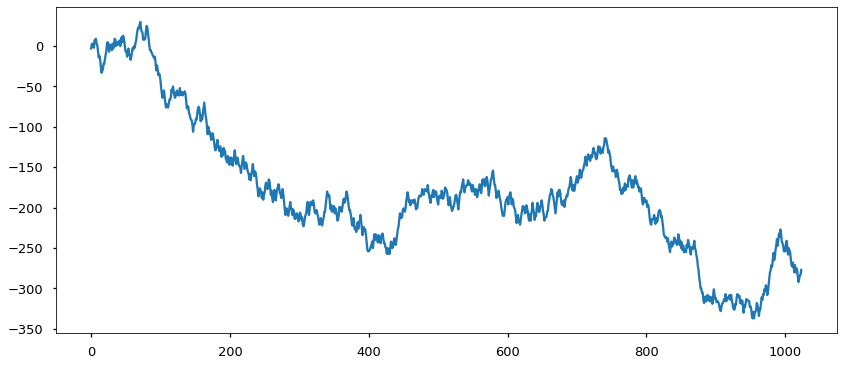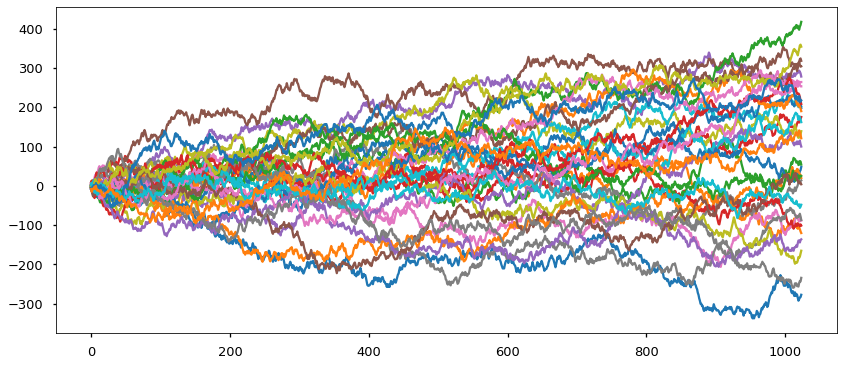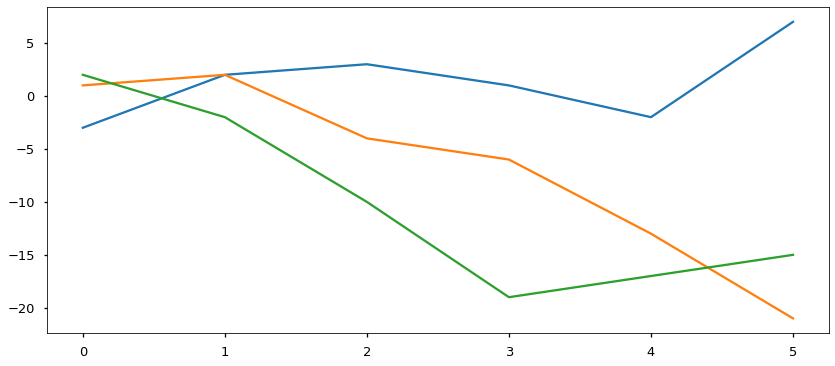# 02.05 Random Walk¶

A random walk is a mathematical concept where random steps are performed repeatedly. Each step consist of adding a random number the the current cumulative sum of all previous steps. Variants of the random walk allow the random numbers to be units (-1 or 1), floating points, limited within a range, and/or taken from a distribution.

Several stochastic processes can be modeled through a random walk, notably processes seen in nature. We will simulate a handful of random walks and get a hang of vectorized computing and simple plotting in the process.

The pyplot is an interface to many plotting facilities in matplotlib. For now do not worry about the %matplotlib inline line.

In :
import numpy as np
import matplotlib.pyplot as plt
%matplotlib inline
plt.style.use('seaborn-talk')np-brownie.svg

## Vectorized Summing¶

We generate a simple random walk by generating random numbers and summing them.

In :
rng = np.random.RandomState(42)
steps = 1024
numbers = rng.randint(-9, 10, steps)
sums = numbers.cumsum()
plt.figure(figsize=(14, 6))
plt.plot(sums);What we did is a loop without actually writing a loop: an aggregation. Yet, we can do better. If we generate a matrix of random numbers we can sum across columns, and build several random walks at once.

In :
rng = np.random.RandomState(42)
steps = 1024
runs = 32
numbers = rng.randint(-9, 10, (runs, steps))
sums = numbers.cumsum(axis=1)
plt.figure(figsize=(14, 6))
plt.plot(sums.T);### Step by Step¶

That was a lot to take in, slow down. Let's reduce the number of steps and walks and do an in-deep look at every piece of that code.

In :
rng = np.random.RandomState(42)
steps = 6
runs = 3
numbers = rng.randint(-9, 10, (runs, steps))
numbers

Out:
array([[-3,  5,  1, -2, -3,  9],
[ 1,  1, -6, -2, -7, -8],
[ 2, -4, -8, -9,  2,  2]])

We have three sets of random numbers, three rows in a matrix.

In :
sums = numbers.cumsum(axis=1)
sums

Out:
array([[ -3,   2,   3,   1,  -2,   7],
[  1,   2,  -4,  -6, -13, -21],
[  2,  -2, -10, -19, -17, -15]])

axis=1 means: perform the aggregation across columns. Therefore we performed a cumulative sum inside each row, we now have three random walks inside each row.

In :
sums.T

Out:
array([[ -3,   1,   2],
[  2,   2,  -2],
[  3,  -4, -10],
[  1,  -6, -19],
[ -2, -13, -17],
[  7, -21, -15]])

The plotting engine understands columns as separate functions, therefore we need to transpose (.T) the matrix for plotting. We only need to call plt.figure to parametrize the image, in this case its size, in inches. The default size of the image is 5 by 5 inches, which is quite small for most uses.

Note: The notebook main area uses a resolution of 72 DPI (dots per inch). Which means that its width resolution of 14-15 inches is just around 1024 pixels. Any image bigger than that (in pixels or inches) will be scaled down, and its aspect ratio adapted accordingly.

In :
plt.figure(figsize=(14, 6))
plt.plot(sums.T);Let's go back to the full example.

In :
rng = np.random.RandomState(42)
steps = 1024
runs = 32
numbers = rng.randint(-9, 10, (runs, steps))
sums = numbers.cumsum(axis=1)
plt.figure(figsize=(14, 6))
plt.plot(sums.T);